# The Convex Geometry of Linear Inverse Problems

## Abstract

In applications throughout science and engineering one is often faced with the challenge of solving an ill-posed inverse problem, where the number of available measurements is smaller than the dimension of the model to be estimated. However in many practical situations of interest, models are constrained structurally so that they only have a few degrees of freedom relative to their ambient dimension. This paper provides a general framework to convert notions of simplicity into convex penalty functions, resulting in convex optimization solutions to linear, underdetermined inverse problems. The class of simple models considered includes those formed as the sum of a few atoms from some (possibly infinite) elementary atomic set; examples include well-studied cases from many technical fields such as sparse vectors (signal processing, statistics) and low-rank matrices (control, statistics), as well as several others including sums of a few permutation matrices (ranked elections, multiobject tracking), low-rank tensors (computer vision, neuroscience), orthogonal matrices (machine learning), and atomic measures (system identification). The convex programming formulation is based on minimizing the norm induced by the convex hull of the atomic set; this norm is referred to as the atomic norm. The facial structure of the atomic norm ball carries a number of favorable properties that are useful for recovering simple models, and an analysis of the underlying convex geometry provides sharp estimates of the number of generic measurements required for exact and robust recovery of models from partial information. These estimates are based on computing the Gaussian widths of tangent cones to the atomic norm ball. When the atomic set has algebraic structure the resulting optimization problems can be solved or approximated via semidefinite programming. The quality of these approximations affects the number of measurements required for recovery, and this tradeoff is characterized via some examples. Thus this work extends the catalog of simple models (beyond sparse vectors and low-rank matrices) that can be recovered from limited linear information via tractable convex programming.

This is a preview of subscription content, access via your institution.

1. 1.

spherical cap is a subset of the sphere obtained by intersecting the sphere $$\mathbb{S}^{p-1}$$ with a halfspace.

2. 2.

While Proposition 3.15 follows as a consequence of the general result in Corollary 3.14, one can remove the constant factor 9 in the statement of Proposition 3.15 by carrying out a more refined analysis of the Birkhoff polytope.

## References

1. 1.

S. Aja-Fernandez, R. Garcia, D. Tao, X. Li, Tensors in Image Processing and Computer Vision. Advances in Pattern Recognition (Springer, Berlin, 2009).

2. 2.

N. Alon, A. Naor, Approximating the cut-norm via Grothendieck’s inequality, SIAM J. Comput. 35, 787–803 (2006).

3. 3.

A. Barron, Universal approximation bounds for superpositions of a sigmoidal function, IEEE Trans. Inf. Theory 39, 930–945 (1993).

4. 4.

A. Barvinok, A Course in Convexity (American Mathematical Society, Providence, 2002).

5. 5.

C. Beckmann, S. Smith, Tensorial extensions of independent component analysis for multisubject FMRI analysis, NeuroImage 25, 294–311 (2005).

6. 6.

D. Bertsekas, Constrained Optimization and Lagrange Multiplier Methods (Athena Scientific, Nashua, 2007).

7. 7.

D. Bertsekas, A. Nedic, A. Ozdaglar, Convex Analysis and Optimization (Athena Scientific, Nashua, 2003).

8. 8.

P. Bickel, Y. Ritov, A. Tsybakov, Simultaneous analysis of Lasso and Dantzig selector, Ann. Stat. 37, 1705–1732 (2009).

9. 9.

J. Bochnak, M. Coste, M. Roy, Real Algebraic Geometry (Springer, Berlin, 1988).

10. 10.

F.F. Bonsall, A general atomic decomposition theorem and Banach’s closed range theorem, Q. J. Math. 42, 9–14 (1991).

11. 11.

A. Brieden, P. Gritzmann, R. Kannan, V. Klee, L. Lovasz, M. Simonovits, Approximation of diameters: randomization doesn’t help, in Proceedings of the 39th Annual Symposium on Foundations of Computer Science (1998), pp. 244–251.

12. 12.

J. Cai, E. Candès, Z. Shen, A singular value thresholding algorithm for matrix completion, SIAM J. Optim. 20, 1956–1982 (2008).

13. 13.

J. Cai, S. Osher, Z. Shen, Linearized Bregman iterations for compressed sensing, Math. Comput. 78, 1515–1536 (2009).

14. 14.

E. Candès, X. Li, Y. Ma, J. Wright, Robust principal component analysis? J. ACM 58, 1–37 (2011).

15. 15.

E. Candès, Y. Plan, Tight oracle inequalities for low-rank matrix recovery from a minimal number of noisy random measurements, IEEE Trans. Inf. Theory 57, 2342–2359 (2011).

16. 16.

E.J. Candès, J. Romberg, T. Tao, Robust uncertainty principles: exact signal reconstruction from highly incomplete frequency information, IEEE Trans. Inf. Theory 52, 489–509 (2006).

17. 17.

E.J. Candès, B. Recht, Exact matrix completion via convex optimization, Found. Comput. Math. 9, 717–772 (2009).

18. 18.

E. Candès, T. Tao, Decoding by linear programming, IEEE Trans. Inf. Theory 51, 4203–4215 (2005).

19. 19.

V. Chandrasekaran, S. Sanghavi, P.A. Parrilo, A.S. Willsky, Rank-sparsity incoherence for matrix decomposition, SIAM J. Optim. 21, 572–596 (2011).

20. 20.

P. Combettes, V. Wajs, Signal recovery by proximal forward-backward splitting, Multiscale Model. Simul. 4, 1168–1200 (2005).

21. 21.

I. Daubechies, M. Defriese, C. De Mol, An iterative thresholding algorithm for linear inverse problems with a sparsity constraint, Commun. Pure Appl. Math. LVII, 1413–1457 (2004).

22. 22.

K.R. Davidson, S.J. Szarek, Local operator theory, random matrices and Banach spaces, in Handbook of the Geometry of Banach Spaces, vol. I (2001), pp. 317–366.

23. 23.

V. de Silva, L. Lim, Tensor rank and the ill-posedness of the best low-rank approximation problem, SIAM J. Matrix Anal. Appl. 30, 1084–1127 (2008).

24. 24.

R. DeVore, V. Temlyakov, Some remarks on greedy algorithms, Adv. Comput. Math. 5, 173–187 (1996).

25. 25.

M. Deza, M. Laurent, Geometry of Cuts and Metrics (Springer, Berlin, 1997).

26. 26.

D.L. Donoho, High-dimensional centrally-symmetric polytopes with neighborliness proportional to dimension, Discrete Comput. Geom. (online) (2005).

27. 27.

D.L. Donoho, For most large underdetermined systems of linear equations the minimal 1-norm solution is also the sparsest solution, Commun. Pure Appl. Math. 59, 797–829 (2006).

28. 28.

D.L. Donoho, Compressed sensing, IEEE Trans. Inf. Theory 52, 1289–1306 (2006).

29. 29.

D. Donoho, J. Tanner, Sparse nonnegative solution of underdetermined linear equations by linear programming, Proc. Natl. Acad. Sci. USA 102, 9446–9451 (2005).

30. 30.

D. Donoho, J. Tanner, Counting faces of randomly-projected polytopes when the projection radically lowers dimension, J. Am. Math. Soc. 22, 1–53 (2009).

31. 31.

D. Donoho, J. Tanner, Counting the faces of randomly-projected hypercubes and orthants with applications, Discrete Comput. Geom. 43, 522–541 (2010).

32. 32.

R.M. Dudley, The sizes of compact subsets of Hilbert space and continuity of Gaussian processes, J. Funct. Anal. 1, 290–330 (1967).

33. 33.

M. Dyer, A. Frieze, R. Kannan, A random polynomial-time algorithm for approximating the volume of convex bodies, J. ACM 38, 1–17 (1991).

34. 34.

M. Fazel, Matrix rank minimization with applications, Ph.D. thesis, Department of Electrical Engineering, Stanford University (2002).

35. 35.

M. Figueiredo, R. Nowak, An EM algorithm for wavelet-based image restoration, IEEE Trans. Image Process. 12, 906–916 (2003).

36. 36.

M. Fukushima, H. Mine, A generalized proximal point algorithm for certain non-convex minimization problems, Int. J. Inf. Syst. Sci. 12, 989–1000 (1981).

37. 37.

M. Goemans, D. Williamson, Improved approximation algorithms for maximum cut and satisfiability problems using semidefinite programming, J. ACM 42, 1115–1145 (1995).

38. 38.

Y. Gordon, On Milman’s inequality and random subspaces which escape through a mesh in ℝn, in Geometric Aspects of Functional Analysis, Israel Seminar 1986–1987. Lecture Notes in Mathematics, vol. 1317 (1988), pp. 84–106.

39. 39.

J. Gouveia, P. Parrilo, R. Thomas, Theta bodies for polynomial ideals, SIAM J. Optim. 20, 2097–2118 (2010).

40. 40.

T. Hale, W. Yin, Y. Zhang, A fixed-point continuation method for 1-regularized minimization: methodology and convergence, SIAM J. Optim. 19, 1107–1130 (2008).

41. 41.

J. Harris, Algebraic Geometry: A First Course (Springer, Berlin).

42. 42.

J. Haupt, W.U. Bajwa, G. Raz, R. Nowak, Toeplitz compressed sensing matrices with applications to sparse channel estimation, IEEE Trans. Inform. Theory 56(11), 5862–5875 (2010).

43. 43.

S. Jagabathula, D. Shah, Inferring rankings using constrained sensing, IEEE Trans. Inf. Theory 57, 7288–7306 (2011).

44. 44.

L. Jones, A simple lemma on greedy approximation in Hilbert space and convergence rates for projection pursuit regression and neural network training, Ann. Stat. 20, 608–613 (1992).

45. 45.

D. Klain, G. Rota, Introduction to Geometric Probability (Cambridge University Press, Cambridge, 1997).

46. 46.

T. Kolda, Orthogonal tensor decompositions, SIAM J. Matrix Anal. Appl. 23, 243–255 (2001).

47. 47.

T. Kolda, B. Bader, Tensor decompositions and applications, SIAM Rev. 51, 455–500 (2009).

48. 48.

M. Ledoux, The Concentration of Measure Phenomenon (American Mathematical Society, Providence, 2000).

49. 49.

M. Ledoux, M. Talagrand, Probability in Banach Spaces (Springer, Berlin, 1991).

50. 50.

J. Löfberg, YALMIP: A toolbox for modeling and optimization in MATLAB, in Proceedings of the CACSD Conference, Taiwan (2004). Available from http://control.ee.ethz.ch/~joloef/yalmip.php.

51. 51.

S. Ma, D. Goldfarb, L. Chen, Fixed point and Bregman iterative methods for matrix rank minimization, Math. Program. 128, 321–353 (2011).

52. 52.

O. Mangasarian, B. Recht, Probability of unique integer solution to a system of linear equations, Eur. J. Oper. Res. 214, 27–30 (2011).

53. 53.

J. Matoušek, Lectures on Discrete Geometry (Springer, Berlin, 2002).

54. 54.

S. Negahban, P. Ravikumar, M. Wainwright, B. Yu, A unified framework for high-dimensional analysis of M-estimators with decomposable regularizers, Preprint (2010).

55. 55.

Y. Nesterov, Quality of semidefinite relaxation for nonconvex quadratic optimization. Technical report (1997).

56. 56.

Y. Nesterov, Introductory Lectures on Convex Optimization (Kluwer Academic, Amsterdam, 2004).

57. 57.

Y. Nesterov, Gradient methods for minimizing composite functions, CORE discussion paper 76 (2007).

58. 58.

P.A. Parrilo, Semidefinite programming relaxations for semialgebraic problems, Math. Program. 96, 293–320 (2003).

59. 59.

G. Pisier, Remarques sur un résultat non publié de B. Maurey. Séminaire d’analyse fonctionnelle (Ecole Polytechnique Centre de Mathematiques, Palaiseau, 1981).

60. 60.

G. Pisier, Probabilistic methods in the geometry of Banach spaces, in Probability and Analysis, pp. 167–241 (1986).

61. 61.

E. Polak, Optimization: Algorithms and Consistent Approximations (Springer, Berlin, 1997).

62. 62.

H. Rauhut, Circulant and Toeplitz matrices in compressed sensing, in Proceedings of SPARS’09, (2009).

63. 63.

B. Recht, M. Fazel, P.A. Parrilo, Guaranteed minimum rank solutions to linear matrix equations via nuclear norm minimization, SIAM Rev. 52, 471–501 (2010).

64. 64.

B. Recht, W. Xu, B. Hassibi, Null space conditions and thresholds for rank minimization, Math. Program., Ser. B 127, 175–211 (2011).

65. 65.

R.T. Rockafellar, Convex Analysis (Princeton University Press, Princeton, 1970).

66. 66.

M. Rudelson, R. Vershynin, Sparse reconstruction by convex relaxation: Fourier and Gaussian measurements, in CISS 2006 (40th Annual Conference on Information Sciences and Systems) (2006).

67. 67.

R. Sanyal, F. Sottile, B. Sturmfels, Orbitopes, Preprint, arXiv:0911.5436 (2009).

68. 68.

N. Srebro, A. Shraibman, Rank, trace-norm and max-norm in 18th Annual Conference on Learning Theory (COLT) (2005).

69. 69.

M. Stojnic, Various thresholds for 1-optimization in compressed sensing, Preprint, arXiv:0907.3666 (2009).

70. 70.

K. Toh, M. Todd, R. Tutuncu, SDPT3—a MATLAB software package for semidefinite-quadratic-linear programming. Available from. http://www.math.nus.edu.sg/~mattohkc/sdpt3.html.

71. 71.

K. Toh, S. Yun, An accelerated proximal gradient algorithm for nuclear norm regularized least squares problems, Pac. J. Optim. 6, 615–640 (2010).

72. 72.

S. van de Geer, P. Bühlmann, On the conditions used to prove oracle results for the Lasso, Electron. J. Stat. 3, 1360–1392 (2009).

73. 73.

S. Wright, R. Nowak, M. Figueiredo, Sparse reconstruction by separable approximation, IEEE Trans. Signal Process. 57, 2479–2493 (2009).

74. 74.

W. Xu, B. Hassibi, Compressive sensing over the Grassmann manifold: a unified geometric framework, IEEE Trans. Inform. Theory 57(10), 6894–6919 (2011).

75. 75.

W. Yin, S. Osher, J. Darbon, D. Goldfarb, Bregman iterative algorithms for compressed sensing and related problems, SIAM J. Imaging Sci. 1, 143–168 (2008).

76. 76.

G. Ziegler, Lectures on Polytopes (Springer, Berlin, 1995).

## Acknowledgements

This work was supported in part by AFOSR grant FA9550-08-1-0180, in part by a MURI through ARO grant W911NF-06-1-0076, in part by a MURI through AFOSR grant FA9550-06-1-0303, in part by NSF FRG 0757207, in part through ONR award N00014-11-1-0723, and NSF award CCF-1139953.

We gratefully acknowledge Holger Rauhut for several suggestions on how to improve the presentation in Sect. 3, and Amin Jalali for pointing out an error in a previous draft. We thank Santosh Vempala, Joel Tropp, Bill Helton, Martin Jaggi, and Jonathan Kelner for helpful discussions. Finally, we acknowledge the suggestions of the associate editor Emmanuel Candès as well as the comments and pointers to references made by the reviewers, all of which improved our paper.

## Author information

Authors

### Corresponding author

Correspondence to Venkat Chandrasekaran.

Communicated by Emmanuel Candès.

## Appendices

### Proof

First note that the Gaussian width can be upper-bounded as follows:

$$w\bigl(\mathcal{C} \cap\mathbb{S}^{p-1}\bigr) \leq \operatorname{\mathbb{E}}_\mathbf{g}\Bigl[ \sup_{\mathbf{z}\in \mathcal{C}\cap\mathcal{B}(0,1)} \mathbf{g}^T \mathbf{z}\Bigr],$$
(30)

where $$\mathcal{B}(0,1)$$ denotes the unit Euclidean ball. The expression on the right-hand side inside the expected value can be expressed as the optimal value of the following convex optimization problem for each g∈ℝp:

$$\begin{array}{l@{\ }l} \displaystyle\max_{\mathbf{z}} & \mathbf{g}^T \mathbf{z}\\[5pt] \mathrm{s.t.} & \mathbf{z}\in\mathcal{C},\\[5pt] & \|\mathbf{z}\|^2\leq1. \end{array}$$
(31)

We now proceed to form the dual problem of (31) by first introducing the Lagrangian

$$\mathcal{L}(\mathbf{z},\mathbf{u},\gamma) = \mathbf{g}^T \mathbf {z}+ \gamma\bigl(1- \mathbf{z}^T \mathbf{z}\bigr) - \mathbf{u}^T \mathbf{z}$$

where $$\mathbf{u}\in\mathcal{C}^{\ast}$$ and γ≥0 is a scalar. To obtain the dual problem we maximize the Lagrangian with respect to z, which amounts to setting

$$\mathbf{z}= \frac{1}{2\gamma} (\mathbf{g}-\mathbf{u}).$$

Putting this into the Lagrangian above gives the dual problem

$$\begin{array}{l@{\ }l} \min& \gamma+ \dfrac{1}{4\gamma} \|\mathbf{g}-\mathbf{u}\|^2\\[7pt] \mathrm{s.t.} & \mathbf{u}\in\mathcal{C}^\ast,\\ & \gamma\geq0. \end{array}$$

Solving this optimization problem with respect to γ we find that $$\gamma= \frac{1}{2} \|\mathbf{g}-\mathbf{u}\|$$, which gives the dual problem to (31)

$$\begin{array}{l@{\ }l} \min& \|\mathbf{g}-\mathbf{u}\|\\[5pt] \mathrm{s.t.} & \mathbf{u}\in\mathcal{C}^\ast. \end{array}$$
(32)

Under very mild assumptions about $$\mathcal{C}$$, the optimal value of (32) is equal to that of (31) (for example as long as $$\mathcal{C}$$ has a nonempty relative interior, strong duality holds). Hence we have derived

$$\operatorname{\mathbb{E}}_\mathbf{g}\Bigl[ \sup_{\mathbf{z}\in\mathcal{C}\cap\mathcal{B}(0,1)} \mathbf{g}^T \mathbf{z}\Bigr] =\operatorname{\mathbb{E}}_\mathbf {g}\bigl[\mathrm{dist}\bigl(\mathbf{g}, \mathcal{C}^\ast\bigr) \bigr].$$
(33)

This equation combined with the bound (30) gives us the desired result. □

### Proof

We set $$\beta= \tfrac{1}{\varTheta}$$. First note that if $$\beta\geq \tfrac{1}{4} \exp\{\tfrac{p}{9}\}$$ then the width bound exceeds $$\sqrt{p}$$, which is the maximal possible value for the width of $$\mathcal{C}$$. Thus, we will assume throughout that $$\beta\leq \tfrac{1}{4} \exp\{\tfrac{p}{9}\}$$.

Using Proposition 3.6 we need to upper-bound the expected distance to the polar cone. Let $$\mathbf{g}\sim\mathcal {N}(0,I)$$ be a normally distributed random vector. Then the norm of g is independent from the angle of g. That is, ∥g∥ is independent from g/∥g∥. Moreover g/∥g∥ is distributed as a uniform sample on $$\mathbb{S}^{p-1}$$, and $$\operatorname{\mathbb{E}}_{\mathbf{g}}[\|\mathbf{g}\|]\leq\sqrt{p}$$. Thus we have

$$\operatorname{\mathbb{E}}_\mathbf{g}\bigl[\mathrm{dist}\bigl (\mathbf{g},\mathcal{C}^\ast\bigr) \bigr] \leq\operatorname{\mathbb{E}}_\mathbf{g}\bigl[\|\mathbf {g}\|\cdot\mathrm{dist}\bigl(\mathbf{g}/\|\mathbf{g}\|, \mathcal{C}^\ast\cap\mathbb{S}^{p-1}\bigr)\bigr]\leq\sqrt{p} \operatorname{\mathbb{E}}_\mathbf{u}\bigl[ \mathrm{dist}\bigl (\mathbf{u}, \mathcal{C}^\ast\cap \mathbb{S}^{p-1}\bigr)\bigr]$$
(34)

where u is sampled uniformly on $$\mathbb{S}^{p-1}$$.

To bound the latter quantity, we will use isoperimetry. Suppose A is a subset of $$\mathbb{S}^{p-1}$$ and B is a spherical cap with the same volume as A. Let N(A,r) denote the locus of all points in the sphere of Euclidean distance at most r from the set A. Let μ denote the Haar measure on $$\mathbb{S}^{p-1}$$ and let μ(A;r) denote the measure of N(A,r). Then spherical isoperimetry states that μ(A;r)≥μ(B;r) for all r≥0 (see, for example, [48, 53]).

Let B now denote a spherical cap with $$\mu(B)=\mu(\mathcal{C}^{\ast}\cap\mathbb{S}^{p-1})$$. Then we have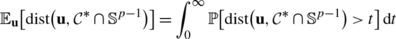(35)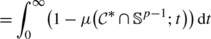(36)(37)

where the first equality is the integral form of the expected value and the last inequality follows by isoperimetry. Hence we can bound the expected distance to the polar cone intersecting the sphere using only knowledge of the volume of spherical caps on $$\mathbb{S}^{p-1}$$.

To proceed let v(φ) denote the volume of a spherical cap subtending a solid angle φ. An explicit formula for v(φ) is

$$v(\varphi)= z_p^{-1}\int_0^\varphi \sin^{p-1}(\vartheta)\,\mathrm{d}\vartheta$$
(38)

where $$z_{p} = \int_{0}^{\pi}\sin^{p-1}(\vartheta)\,\mathrm{d}\vartheta$$ . Let φ(β) denote the minimal solid angle of a cap such that β copies of that cap cover $$\mathbb {S}^{p-1}$$. Since the geodesic distance on the sphere is always greater than or equal to the Euclidean distance, if K is a spherical cap subtending ψ radians, μ(K;t)≥v(ψ+t). Therefore

$$\int_0^\infty\bigl(1- \mu(B;t)\bigr)\,\mathrm{d}t \leq\int_0^\infty\bigl(1-v\bigl( \varphi(\beta)+t\bigr)\bigr)\,\mathrm{d}t .$$
(39)

We can proceed to simplify the right-hand side integral:(40)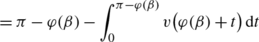(41)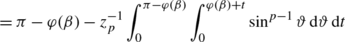(42)(43)(44)(45)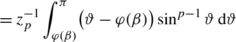(46)

(43) follows by switching the order of integration, and the rest of these equalities follow by straightforward integration and some algebra.

Using the inequalities that $$z_{p} \geq\frac{2}{\sqrt{p-1}}$$ (see ) and sin(x)≤exp(−(xπ/2)2/2) for x∈[0,π], we can bound the last integral as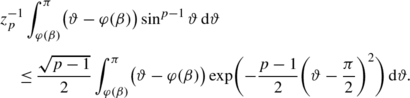(47)

Performing the change of variables $$a = \sqrt{p-1}(\vartheta-\tfrac {\pi}{2})$$, we are left with the integral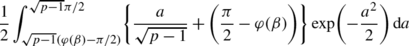(48)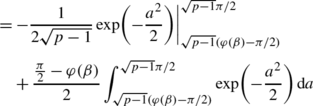(49)(50)

In this final bound, we bounded the first term by dropping the upper integrand, and for the second term we used the fact that

$$\int_{-\infty}^\infty\exp\bigl(-x^2/2\bigr) \,\mathrm{d}x = \sqrt{2\pi}.$$
(51)

We are now left with the task of computing a lower bound for φ(β). We need to first reparameterize the problem. Let K be a spherical cap. Without loss of generality, we may assume that

$$K = \bigl\{ x\in\mathbb{S}^{p-1}:x_1\geq h\bigr\}$$
(52)

for some h∈[0,1]. Here h is the height of the cap over the equator. Via elementary trigonometry, the solid angle that K subtends is given by π/2−sin−1(h). Hence, if h(β) is the largest number such that β caps of height h cover $$\mathbb {S}^{p-1}$$, then h(β)=sin(π/2−φ(β)).

The quantity h(β) may be estimated using the following estimate from . For h∈[0,1], let γ(p,h) denote the volume of a spherical cap of $$\mathbb{S}^{p-1}$$ of height h.

### Lemma B.1

(See )

For $$1\geq h\geq\frac{2}{\sqrt{p}}$$,

$$\frac{1}{10 h \sqrt{p}}\bigl(1-h^2\bigr)^{\frac{p-1}{2}} \leq \gamma(p,h) \leq\frac{1}{2 h \sqrt{p}}\bigl(1-h^2\bigr)^{\frac{p-1}{2}} .$$
(53)

Note that for $$h \geq\frac{2}{\sqrt{p}}$$,

$$\frac{1}{2 h \sqrt{p}}\bigl(1-h^2\bigr)^{\frac{p-1}{2}} \leq \frac{1}{4}\bigl(1-h^2\bigr)^{\frac{p-1}{2}} \leq \frac{1}{4}\exp\biggl(-\frac{p-1}{2} h^2\biggr).$$
(54)

So if

$$h = \sqrt{\frac{2\log(4\beta)}{p-1}}$$
(55)

then h≤1 because we have assumed $$\beta\leq\tfrac{1}{4} \exp\{ \tfrac{p}{9}\}$$ and p≥9. Moreover, $$h\geq\frac{2}{\sqrt{p}}$$ and the volume of the cap with height h is less than or equal to 1/β. That is

$$\varphi(\beta)\geq\pi/2 - \sin^{-1} \biggl( \sqrt{\frac{2\log (4\beta)}{p-1}} \biggr).$$
(56)

Combining the estimate (50) with Proposition 3.6, and using our estimate for φ(β), we get the bound(57)

This expression can be simplified by using the following bounds. First, sin−1(x)≥x lets us upper-bound the first term by $$\sqrt{\frac{p}{p-1}}\frac{1}{8\beta}$$. For the second term, using the inequality $$\sin^{-1}(x)\leq\tfrac{\pi}{2}x$$ results in the upper bound

$$w(\mathcal{C}) \leq\sqrt{\frac{p}{p-1}} \biggl( \frac{1}{8\beta} + \frac{\pi^{3/2}}{2} \sqrt{\log(4\beta)} \biggr).$$
(58)

For p≥9 the upper bound can be expressed simply as $$w(\mathcal{C})\leq3\sqrt{\log(4 \beta)}$$. We recall that $$\beta= \tfrac{1}{\varTheta}$$, which completes the proof of the theorem.  □

### Appendix C: Direct Width Calculations

We first give the proof of Proposition 3.10.

### Proof

Let x be an s-sparse vector in ℝp with 1 norm equal to 1, and let $$\mathcal{A}$$ denote the set of unit-Euclidean-norm one-sparse vectors. Let Δ denote the set of coordinates where x is nonzero. The normal cone at x with respect to the 1 ball is given by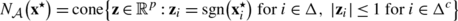(59)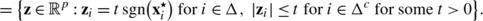(60)

Here Δc represents the zero entries of x . The minimum squared distance to the normal cone at x can be formulated as a one-dimensional convex optimization problem for arbitrary z∈ℝp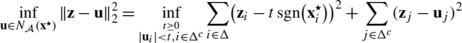(61)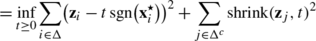(62)

where

$$\mathrm{shrink}(z,t) = \left\{ \begin{array}{l@{\quad}l} z+t & z<-t,\\ 0 & -t\leq z \leq t,\\ z - t & z>t \end{array} \right.$$
(63)

is the 1-shrinkage function. Hence, for any fixed t≥0 independent of g, we have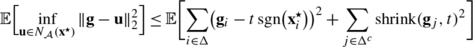(64)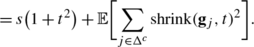(65)

Now we directly integrate the second term, treating each summand individually. For a zero-mean, unit-variance normal random variable g,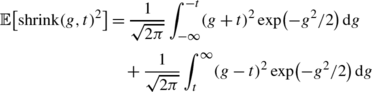(66)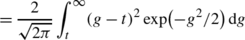(67)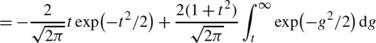(68)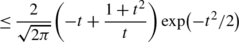(69)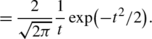(70)

The first simplification follows because the shrink function and Gaussian distributions are symmetric about the origin. The second equality follows by integrating by parts. The inequality follows by a tight bound on the Gaussian Q-function:

$$Q(x) = \frac{1}{\sqrt{2\pi}} \int_{x}^{\infty} \exp \bigl(-g^2/2\bigr) \,\mathrm{d}g \leq\frac{1}{\sqrt{2\pi}}\frac {1}{x}\exp \bigl(-x^2/2\bigr)\quad\mbox{for }x>0.$$
(71)

Using this bound, we get

$$\operatorname{\mathbb{E}}\Bigl[\inf_{\mathbf {u}\in N_\mathcal{A}(\mathbf{x}^{\star})} \|\mathbf{g}-\mathbf{u}\|_2^2 \Bigr] \leq s\bigl(1+t^2\bigr) +(p-s)\frac{2}{\sqrt{2\pi}} \frac{1}{t}\exp\bigl(-t^2/2\bigr).$$
(72)

Setting $$t= \sqrt{2\log(p/s)}$$ gives

$$\operatorname{\mathbb{E}}\Bigl[\inf _{\mathbf{z}\in N_\mathcal{A}(\mathbf{x}^{\star})} \|\mathbf {g}-\mathbf{z} \|_2^2 \Bigr] \leq s \biggl(1+2\log\biggl( \frac{p}{s} \biggr) \biggr)+\frac{s(1-s/p)}{\pi\sqrt{\log (p/s)}}\leq2s\log(p/s )+ \frac{5}{4}s.$$
(73)

The last inequality follows because

$$\frac{(1-s/p)}{\pi\sqrt{\log(p/s)}}\leq0.204<1/4$$
(74)

whenever 0≤sp. □

Next we give the proof of Proposition 3.11.

### Proof

Let x be an m 1×m 2 matrix of rank r with singular value decomposition UΣV , and let $$\mathcal{A}$$ denote the set of rank-one unit-Euclidean-norm matrices of size m 1×m 2. Without loss of generality, impose the conventions m 1m 2, Σ is r×r, U is m 1×r, and V is m 2×r, and assume the nuclear norm of x is equal to 1.

Let u k (respectively v k ) denote the k’th column of U (respectively V). It is convenient to introduce the orthogonal decomposition $${\mathbb{R}}^{m_{1} \times m_{2}} = \Delta\oplus\Delta ^{\perp}$$ where Δ is the linear space spanned by elements of the form u k z T and $$\mathbf{y}\mathbf{v}_{k}^{T}$$, 1≤kr, where z and y are arbitrary, and Δ is the orthogonal complement of Δ. The space Δ is the subspace of matrices spanned by the family (yz T), where y (respectively z) is any vector orthogonal to all the columns of U (respectively V). The normal cone of the nuclear norm ball at x is given by the cone generated by the subdifferential at x :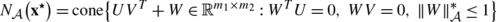(75)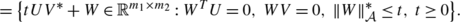(76)

Note that here $$\|Z\|_{\mathcal{A}}^{\ast}$$ is the operator norm, equal to the maximum singular value of Z .

Let G be a Gaussian random matrix with i.i.d. entries, each with mean zero and unit variance. Then the matrix

$$Z(G) = \bigl\|\mathcal{P}_{\Delta^{\perp}}(G)\bigr\| UV^* + \mathcal{P}_{\Delta^{\perp}}(G)$$
(77)

is in the normal cone at x . We can then compute(78)(79)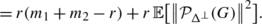(80)

Here (79) follows because $$\mathcal{P}_{\Delta }(G)$$ and $$\mathcal{P}_{\Delta^{\perp}}(G)$$ are independent. The final line follows because dim(T)=r(m 1+m 2r) and the Frobenius (i.e., Euclidean) norm of UV is $$\|UV^{*}\|_{F}=\sqrt{r}$$. Due to the isotropy of Gaussian random matrices, $$\mathcal{P}_{\Delta^{\perp}}(G)$$ is identically distributed as an (m 1r)×(m 2r) matrix with i.i.d. Gaussian entries each with mean zero and variance one. We thus know that

$$\operatorname{\mathbb{P}}\bigl[ \bigl\|\mathcal{P}_{\Delta^{\perp }}(G)\bigr\|\geq\sqrt{m_1-r}+\sqrt {m_2-r} +s \bigr] \leq\exp\bigl(-s^2/2 \bigr)$$
(81)

(see, for example, ). To bound the latter expectation, we again use the integral form of the expected value. Letting $$\mu_{T^{\perp}}$$ denote the quantity $$\sqrt{m_{1}-r}+\sqrt{m_{2}-r}$$, we have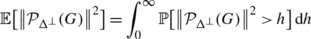(82)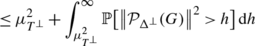(83)(84)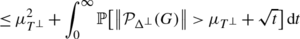(85)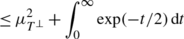(86)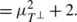(87)

Using this bound in (80), we obtain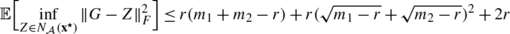(88)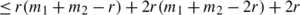(89)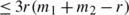(90)

where the second inequality follows from the fact that (a+b)2≤2a 2+2b 2. We conclude that 3r(m 1+m 2r) random measurements are sufficient to recover a rank r, m 1×m 2 matrix using the nuclear norm heuristic. □

## Rights and permissions

Reprints and Permissions

Chandrasekaran, V., Recht, B., Parrilo, P.A. et al. The Convex Geometry of Linear Inverse Problems. Found Comput Math 12, 805–849 (2012). https://doi.org/10.1007/s10208-012-9135-7

• Revised:

• Accepted:

• Published:

• Issue Date:

### Keywords

• Convex optimization
• Semidefinite programming
• Atomic norms
• Real algebraic geometry
• Gaussian width
• Symmetry

• 52A41
• 90C25
• 90C22
• 60D05
• 41A45